Скачать презентацию Understanding How The Buyer Makes Purchasing Decisions

ffb68e9a8ebb7249c826204c6f7ea779.ppt

• Количество слайдов: 9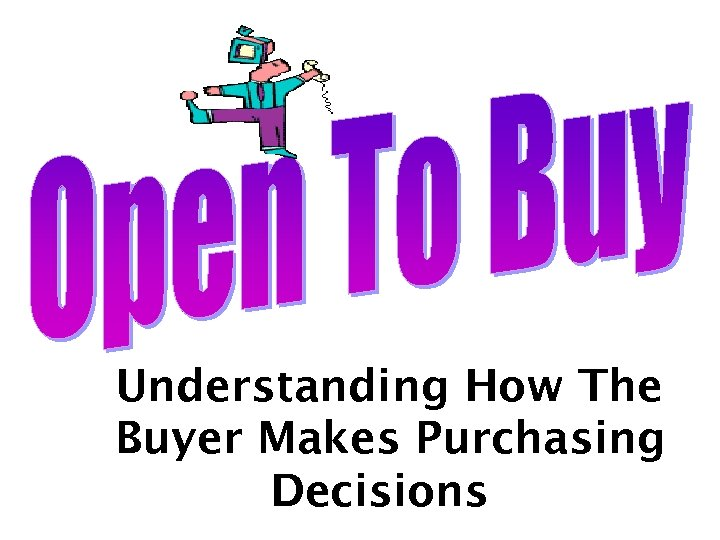Understanding How The Buyer Makes Purchasing Decisions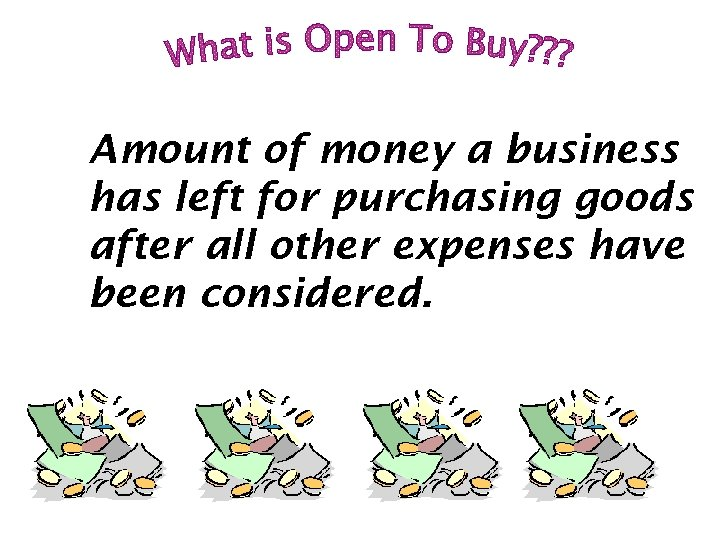Amount of money a business has left for purchasing goods after all other expenses have been considered.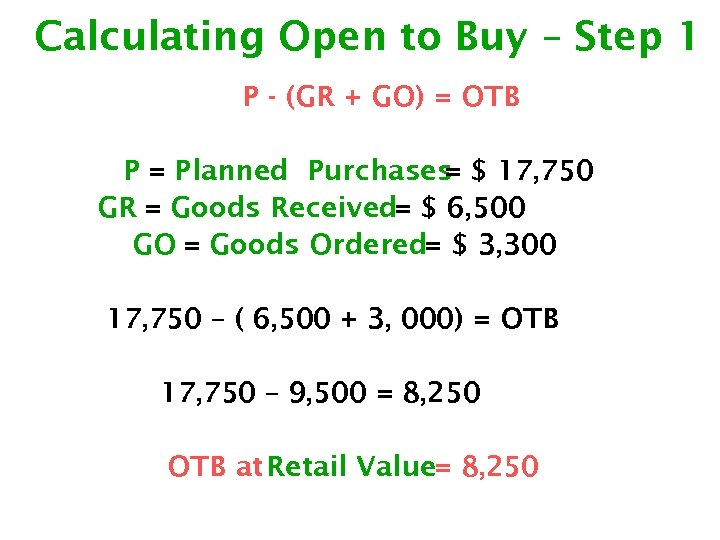Calculating Open to Buy – Step 1 P - (GR + GO) = OTB P = Planned Purchases \$ 17, 750 = GR = Goods Received= \$ 6, 500 GO = Goods Ordered= \$ 3, 300 17, 750 – ( 6, 500 + 3, 000) = OTB 17, 750 – 9, 500 = 8, 250 OTB at Retail Value= 8, 250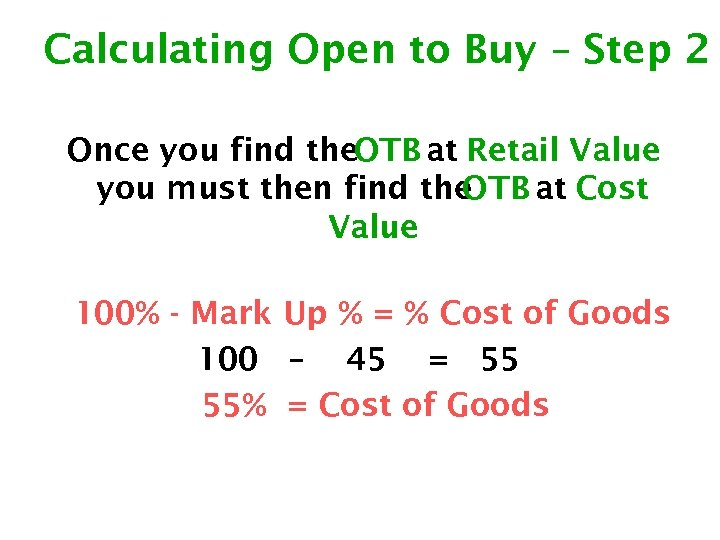Calculating Open to Buy – Step 2 Once you find the. OTB at Retail Value you must then find the OTB at Cost Value 100% - Mark Up % = % Cost of Goods 100 – 45 = 55 55% = Cost of Goods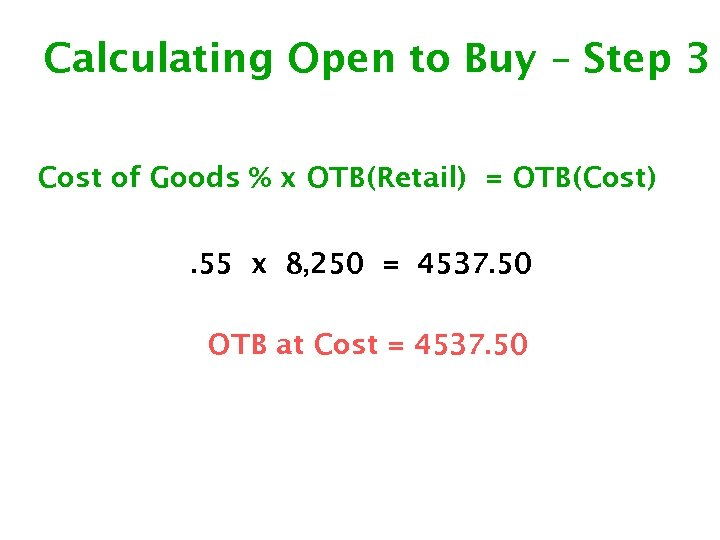Calculating Open to Buy – Step 3 Cost of Goods % x OTB(Retail) = OTB(Cost). 55 x 8, 250 = 4537. 50 OTB at Cost = 4537. 50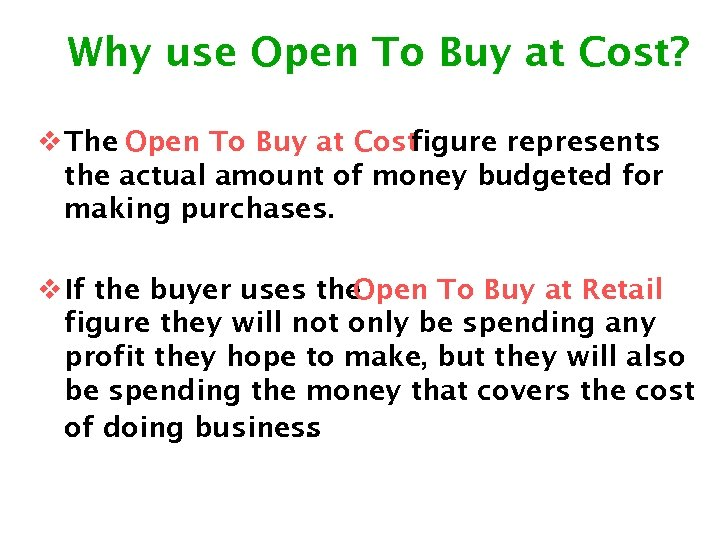Why use Open To Buy at Cost? v The Open To Buy at Cost figure represents the actual amount of money budgeted for making purchases. v If the buyer uses the Open To Buy at Retail figure they will not only be spending any profit they hope to make, but they will also be spending the money that covers the cost of doing business.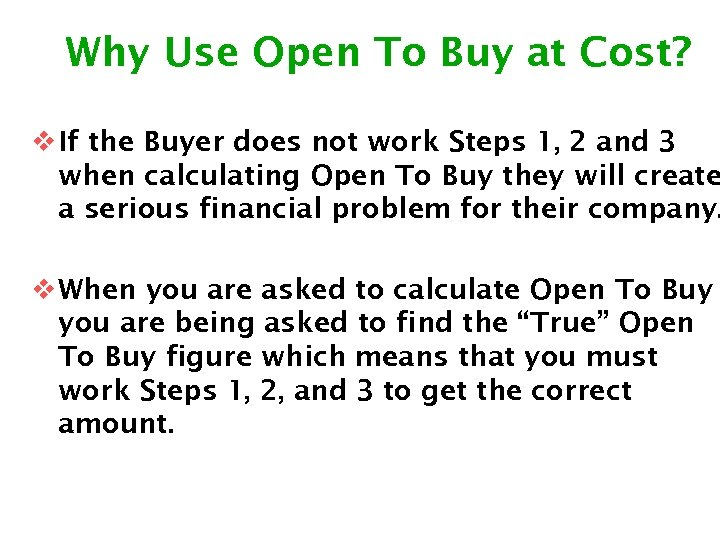Why Use Open To Buy at Cost? v If the Buyer does not work Steps 1, 2 and 3 when calculating Open To Buy they will create a serious financial problem for their company. v When you are asked to calculate Open To Buy you are being asked to find the “True” Open To Buy figure which means that you must work Steps 1, 2, and 3 to get the correct amount.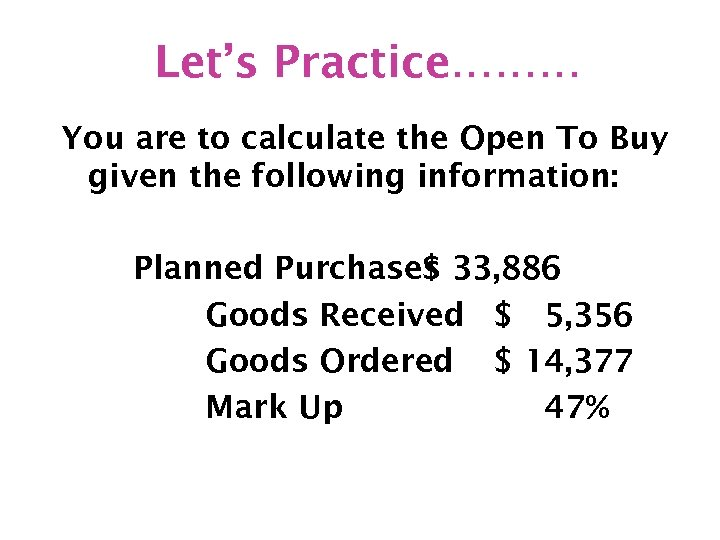Let’s Practice……… You are to calculate the Open To Buy given the following information: Planned Purchases 33, 886 \$ Goods Received \$ 5, 356 Goods Ordered \$ 14, 377 Mark Up 47%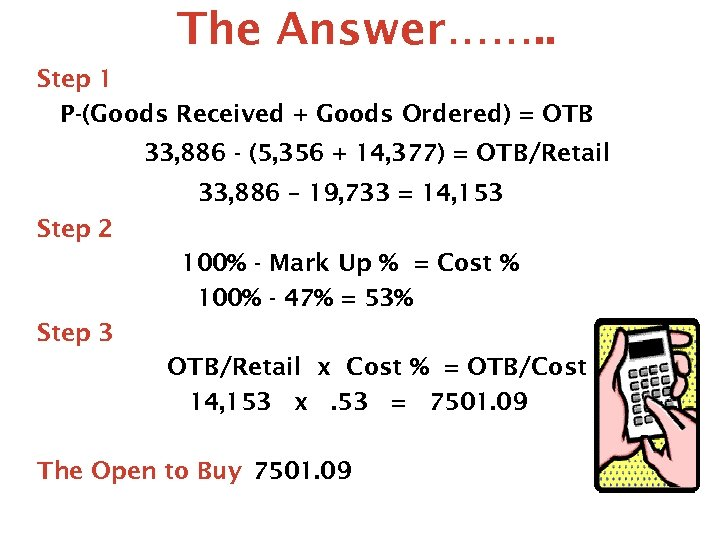The Answer……. . Step 1 P-(Goods Received + Goods Ordered) = OTB 33, 886 - (5, 356 + 14, 377) = OTB/Retail 33, 886 – 19, 733 = 14, 153 Step 2 100% - Mark Up % = Cost % 100% - 47% = 53% Step 3 OTB/Retail x Cost % = OTB/Cost 14, 153 x. 53 = 7501. 09 The Open to Buy 7501. 09